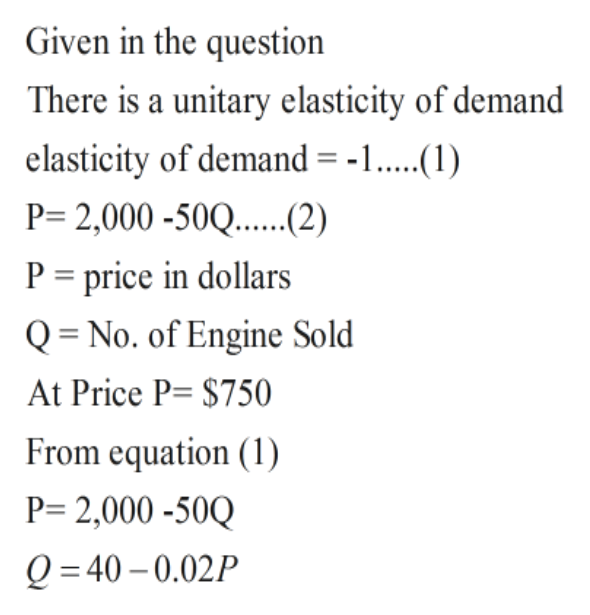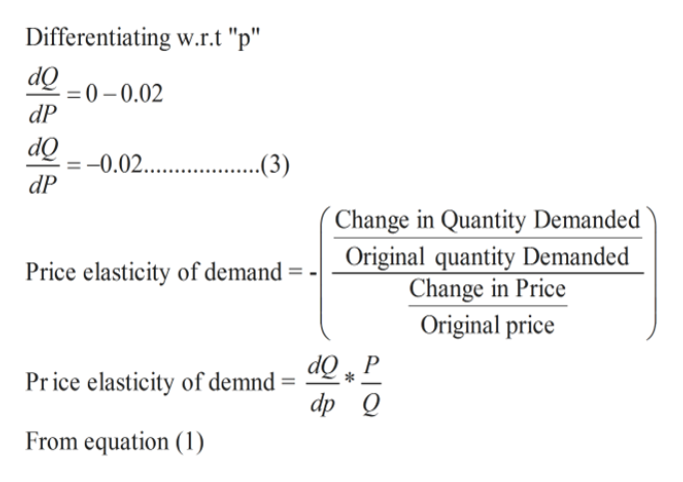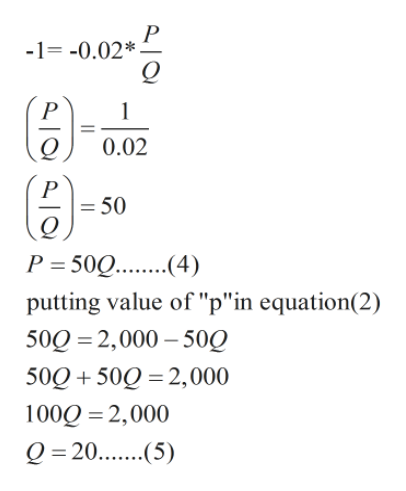# For problems 1 – 4: The Dolan Corporation, a maker of small engines, determines that in 2019 the demand curve for its product is P = 2,000 - 50Q where P is the price (in dollars) of an engine and Q is the number of engines sold per month. At what price, if any, will the demand for Dolan’s engines be of unitary elasticity? a.\$500 b.\$1000 c.\$250 d.\$750

Question
90 views

For problems 1 – 4: The Dolan Corporation, a maker of small engines, determines that in 2019 the demand curve for its product is

= 2,000 - 50Q

where is the price (in dollars) of an engine and is the number of engines sold per month.

At what price, if any, will the demand for Dolan’s engines be of unitary elasticity?

 a. \$500 b. \$1000 c. \$250 d. \$750
check_circle

Step 1

Solution-help_outlineImage TranscriptioncloseGiven in the question There is a unitary elasticity of demand elasticity of demand = -1. P= 2,000 -50Q....2) P price in dollars Q = No. of Engine Sold At Price P= \$750 From equation (1) P 2,000-50Q Q =40 0.02P fullscreen
Step 2help_outlineImage TranscriptioncloseDifferentiating w.r.t "p" dO 0-0.02 dP dQ -0.02 ..(3) dP Change in Quantity Demanded Original quantity Demanded Change in Price Price elasticity of demand Original price Price elasticity of demnd dp Q From equation (1) fullscreen
Step 3help_outlineImage Transcriptionclose-1-0.02* P 0.02 P = 50 P 50Q..4) putting value of "p"in equation(2) 500 2,000 50Q 50Q 50Q 2,000 100Q 2,000 Q =20.(5) fullscreen

### Want to see the full answer?

See Solution

#### Want to see this answer and more?

Solutions are written by subject experts who are available 24/7. Questions are typically answered within 1 hour.*

See Solution
*Response times may vary by subject and question.
Tagged in